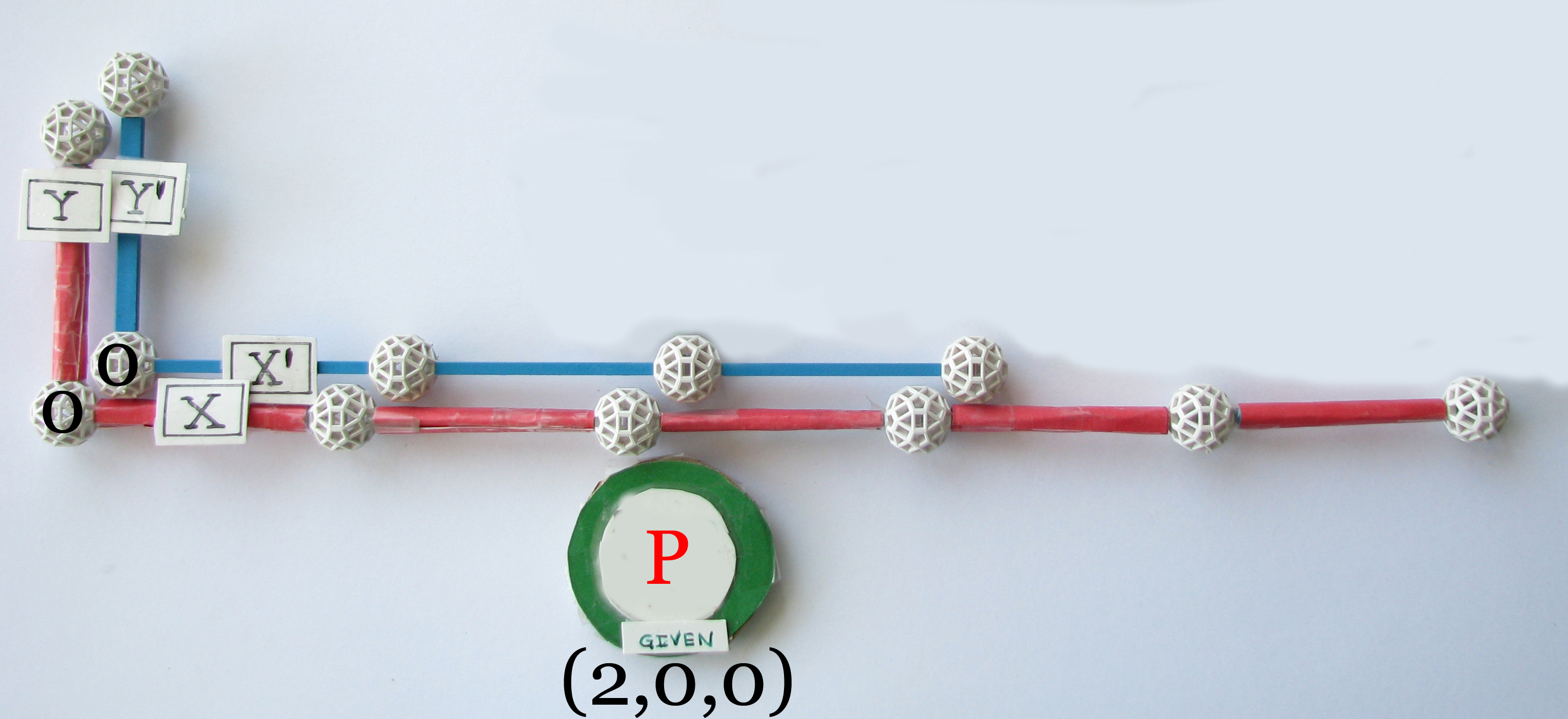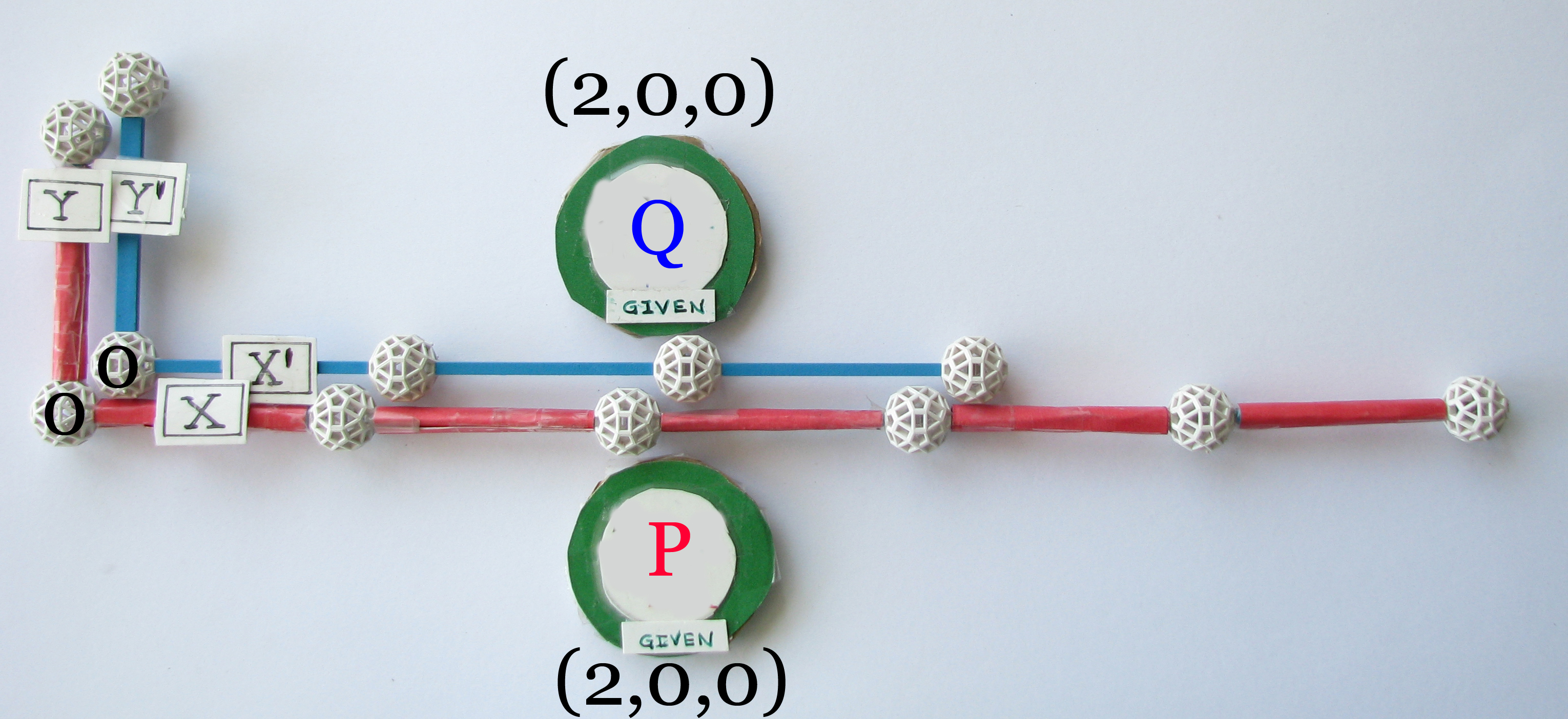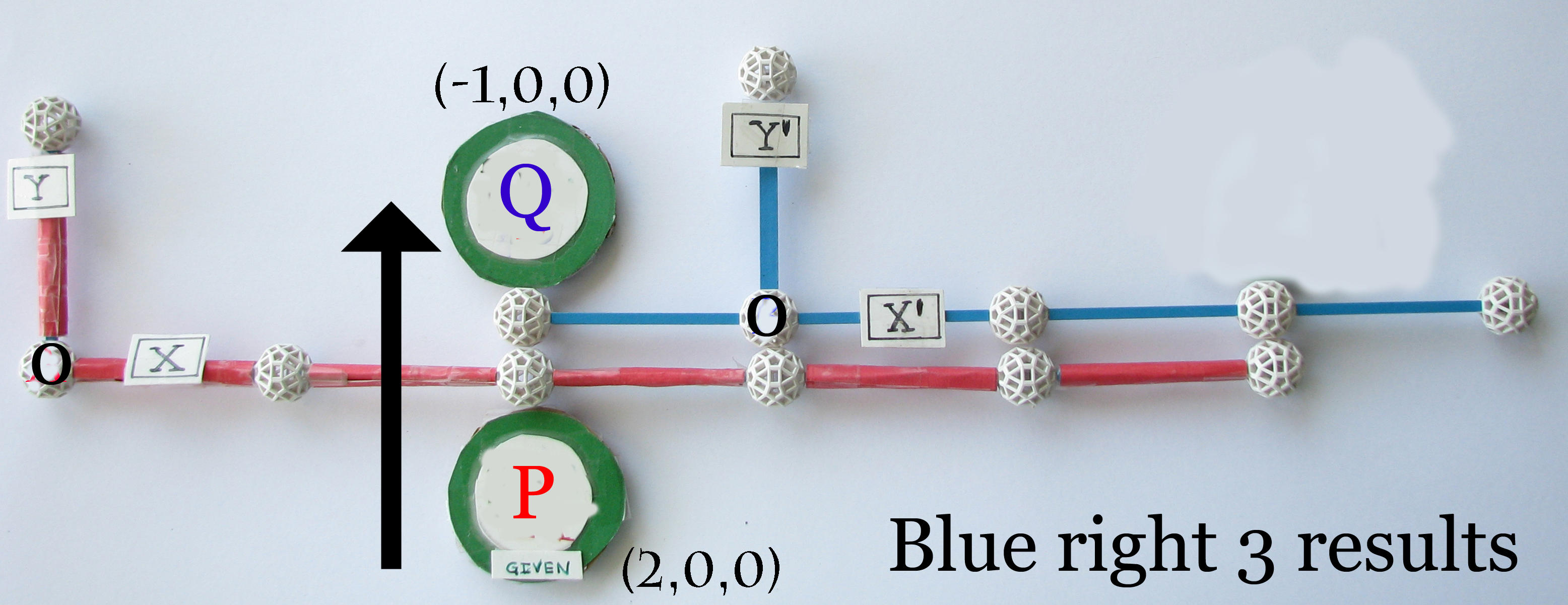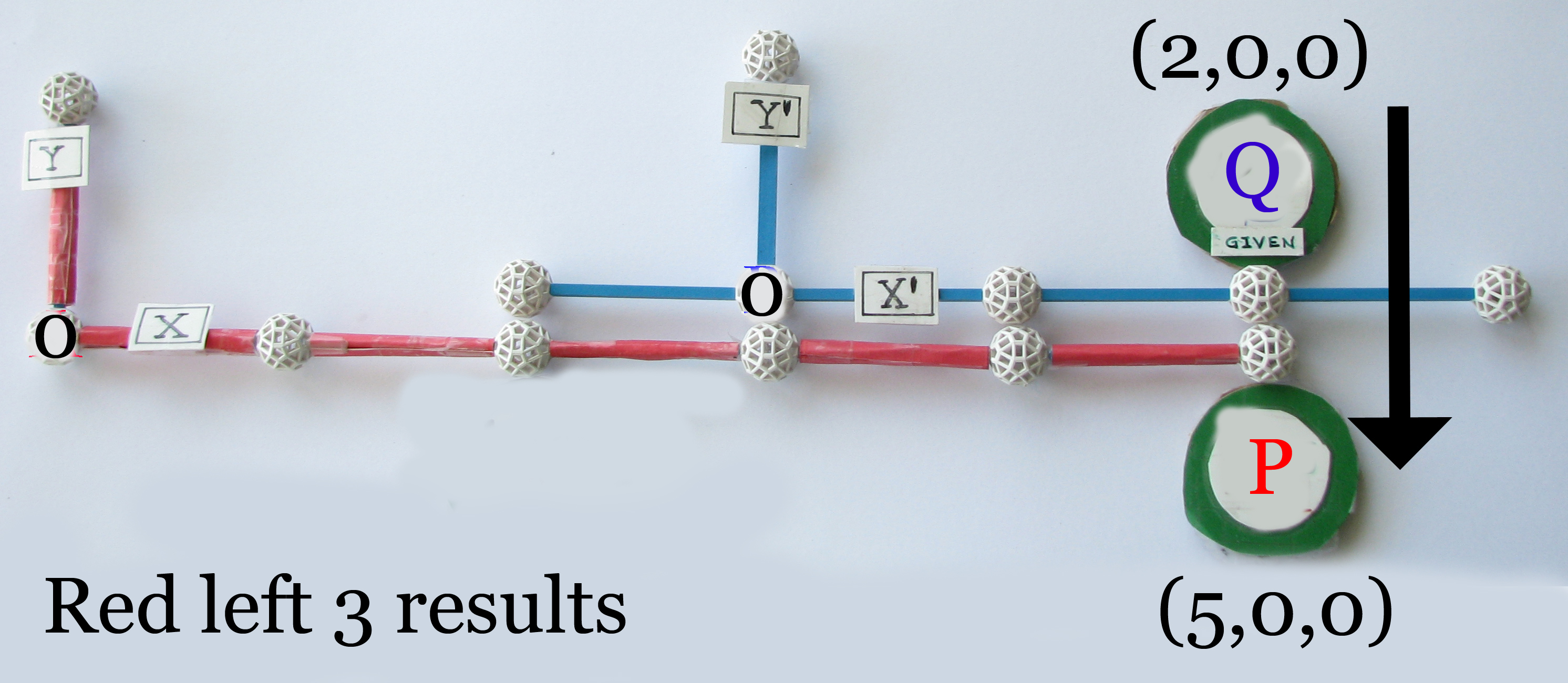coincident coordinate systems...3 question quiz 1 is it mathematically acceptable, to have a selected point in one coincident system and not both simultaneously?yes nogiven coincident systems Red with selected point P at (2,0,0) and selected point Q in Blue (2,0,0)DEFINED RELOCATION occurs when EITHER coincident system moves from mutual coincidence. 2 should the results of the Blue coordinate system relocated/moved right 3 along x' be the same results as if Red system was instead relocated/moved left 3 along xyes no DEFINED TRANSFORMATION has occurred when all SELECTED points in each system are inserted into the opposite system. 3 Given the above depiction, do these two selected point transformations between systems; the Red system left 3 or the Blue system right 3...share identical transformations outcomes?if B right 3, then P in Red = (2,0,0) and Q in Blue = (-1,0,0)if A left 3, then P in Red = (5,0,0) and Q in Blue = (2,0,0) yes no all rights reserved steve waterman April 2012